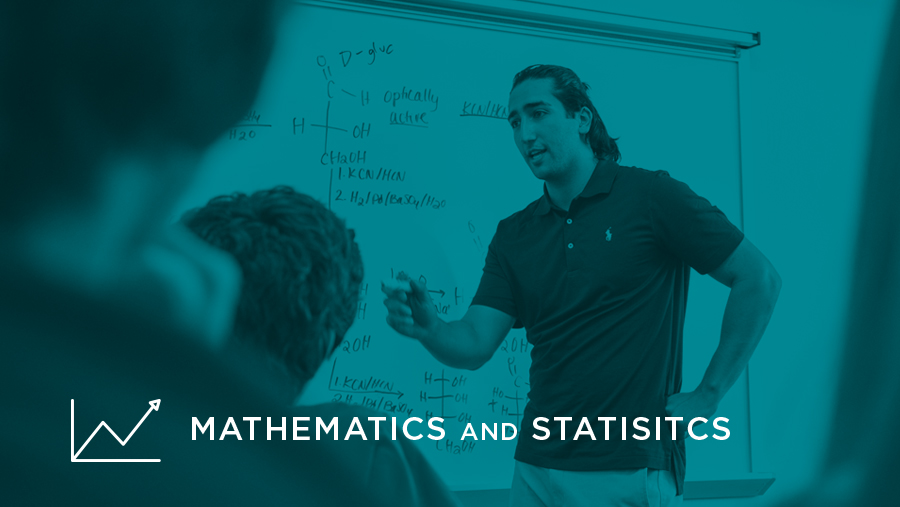# Mathematics and Statistics## Essential Skills:

• Communication
• Quantitative Reasoning
• Critical Thinking

## 3 credit hours:

One course from the list below at the appropriate level, determined by placement.

I prove a theorem and the house expands:
The windows jerk free to hover near the ceiling,
The ceiling floats away with a sigh.

Rita Dove, "Geometry"
• Explore how math and data provide information about the world.
• Explain and present information in mathematical forms.
• Design problems, consider limitations, and calculate results.
• Identify mathematical approaches to address questions.

## Courses

Yucca symbol = This course also meets the U.S. Global Diversity requirement.

Course NumberNameDescription
HNRS 2221Mathematics in the World
MATH 1130Survey of Mathematics
MATH 1220College Algebra
MATH 1240Pre-Calculus
MATH 1250Trigonometry & Pre-Calculus
MATH 1300Statistical Literacy
MATH 1350Introduction to Statistics
MATH 1430Applications of Calculus I
MATH 1440Applications of Calculus II
MATH 1512Calculus 1
MATH 1522Calculus 2
MATH 2118Mathematics for Elementary and Middle School Teachers III